## 【C++心愿便利店】No.3---内联函数、auto、范围for、nullptr

article2023/10/1 11:22:17/

### 文章目录

• 前言
• 🌟一、内联函数
• 🌏1.1.面试题
• 🌏1.2.内联函数概念
• 🌏1.3.内联函数特性
• 🌟二、auto关键字
• 🌏2.1.类型别名思考
• 🌏2.2.auto简介
• 🌏2.3.auto的使用细节
• 🌏2.4.auto不能推导的场景
• 🌏2.5.小场景补充
• 🌟三、基于范围的for循环
• 🌏3.1.范围for的语法
• 🌏3.2.范围for的使用条件
• 🌟四、指针空值nullptr

# 前言👧个人主页：@小沈YO.
😚小编介绍：欢迎来到我的乱七八糟小星球🌝
📋专栏：C++ 心愿便利店
🔑本章内容：内联函数、auto、范围for、nullptr

`提示：以下是本篇文章正文内容，下面案例可供参考`

# 🌟一、内联函数

## 🌏1.1.面试题

``````#define N 10//宏常量
//宏函数
#define ADD(int x , int y) {return x+y;}//宏的调用不需要return
#define ADD(x , y) (return x+y;)
#define ADD(x , y) return x+y;

//加分号是可以的但对于有些语法是不通过的
int main()
{
return 0;
}

int main()
{
return 0;
}

int main()
{
int a = 1, b = 2;
ADD(a | b, a & b);//替换后变成(a|b+a&b)  +号的优先级高于| &所以会先算+
return 0;
}

#define ADD(x , y) ((x)+(y))   这是正确的
``````

1.增强代码的复用性。
2.提高性能。

1.不方便调试宏。（因为预编译阶段进行了替换）
2.导致代码可读性差，可维护性差，容易误用，语法很坑。
3.没有类型安全的检查 。

1.没有类型的严格限制
2.针对频繁调用小函数，不需要再建立栈帧，提高了效率

``````int Add(int left, int right)
//这种函数调用是需要建立栈帧的但是宏函数不需要直接替换了
{
return left + right;
}
``````

C++有哪些技术替代宏？

1. 常量定义 换用const enum
2. 短小函数定义 换用内联函数

## 🌏1.2.内联函数概念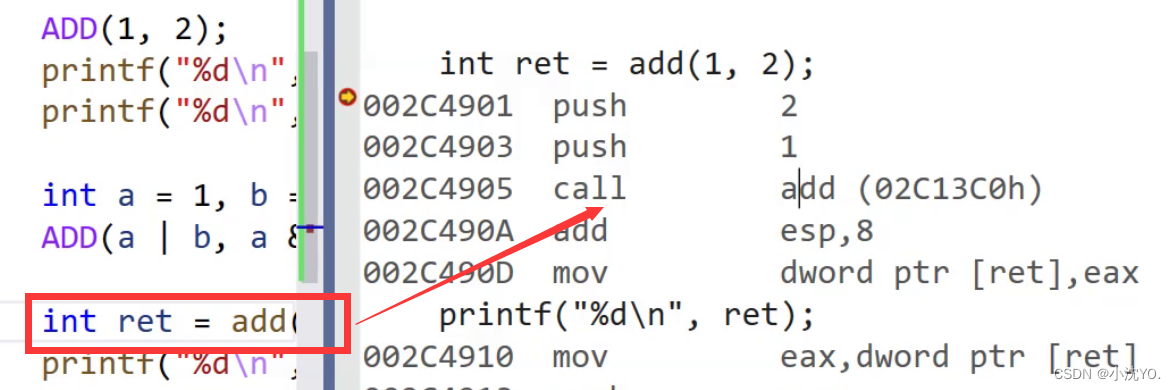2. 在debug模式下，需要对编译器进行设置，否则不会展开(因为debug模式下，编译器默认不会对代码进行优化，以下给出vs2013的设置方式)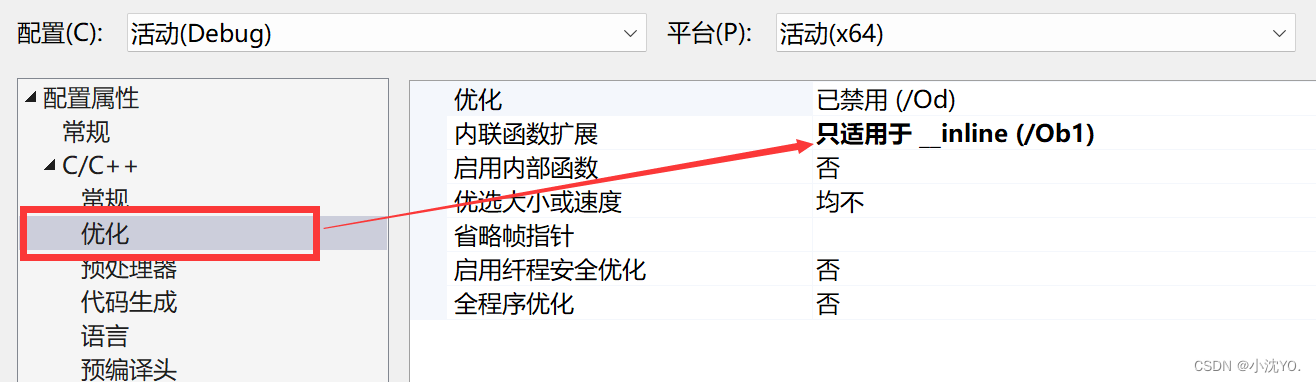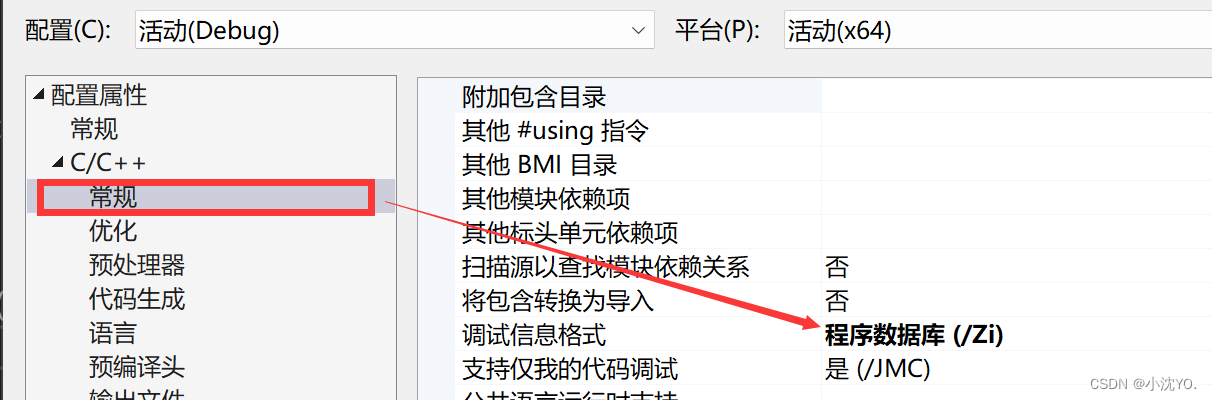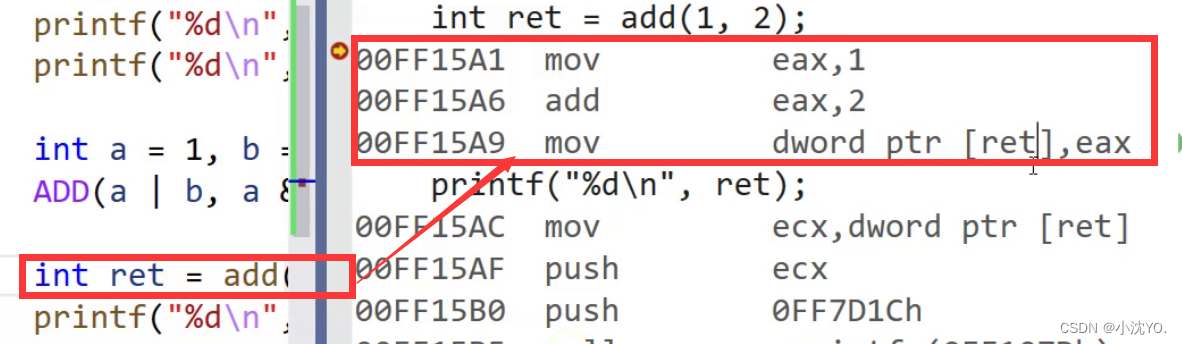``````inline int add(int x, int y)
{
return x + y;
}
int main()
{
int a = 1, b = 2;

//int ret = add(a | b , a & b);
//这样写也不会像宏函数一样出错了
printf("%d\n", ret);
return 0;
}
``````

## 🌏1.3.内联函数特性

inline是一种以空间换时间的做法，如果编译器将函数当成内联函数处理，在编译阶段，会用函数体替换函数调用，缺陷：可能会使目标文件变大（代码膨胀），优势：少了调用开销，提高程序运行效率。（不能任何情况下都用内联）

inline对于编译器而言只是一个建议，不同编译器关于inline实现机制可能不同，一般建议：将函数规模较小(即函数不是很长，具体没有准确的说法，取决于编译器内部实现)、不是递归、且频繁调用的函数采用inline修饰，否则编译器会忽略inline特性。下图为《C++prime》第五版关于inline的建议：

``````inline int add(int x, int y)
{
return x + y;
}
inline int func()
{
int x1= 0;
int x2 = 0;
int x3 = 0;
int x4 = 0;
int ret = 0;
ret += x1;
ret *= x2;
ret /= x3;
ret /= x3;
ret /= x3;
ret += x1;
ret += x1;
return ret;
}
int main()
{
int a = 1, b = 2;
int ret = add(a | b , a & b);
printf("%d\n", ret);
ret = func();
return 0;
}
``````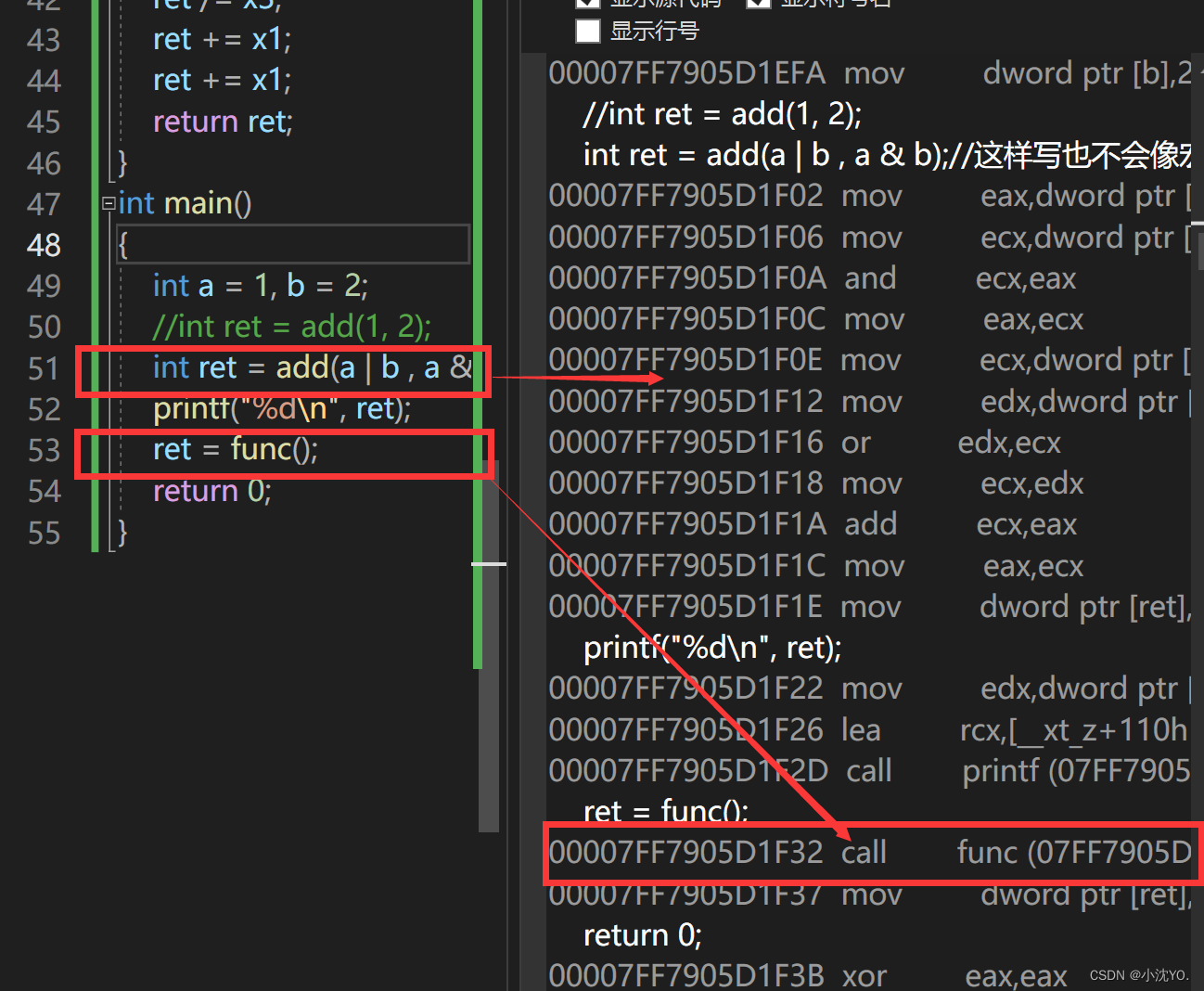``````inline int add(int x, int y)
{
return x + y;
}
inline int func()
{
int x1= 0;
int x2 = 0;
int x3 = 0;
int x4 = 0;
int ret = 0;
ret += x1;
return ret;
}
int main()
{
int a = 1, b = 2;
int ret = add(a | b , a & b);//这样写也不会像宏函数一样出错了
printf("%d\n", ret);
ret = func();
return 0;
}
``````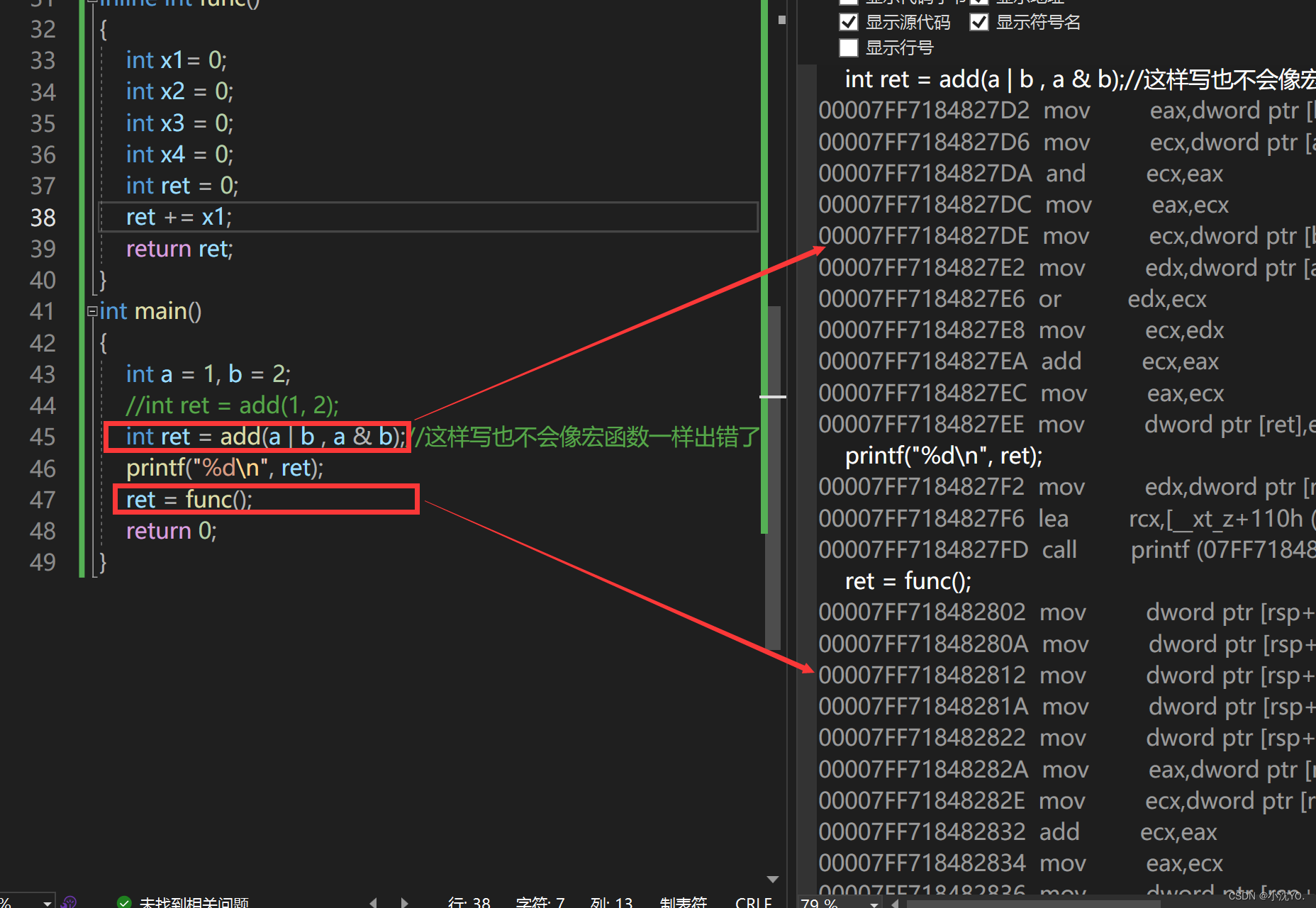inline不建议声明和定义分离，分离会导致链接错误。因为inline被展开就没有函数地址了，链接就会找不到

``````//Func.h
#define _CRT_SECURE_NO_WARNINGS 1
#include<iostream>

using namespace std;

inline void f(int i);

//Func.cpp
#define _CRT_SECURE_NO_WARNINGS 1
#include "Func.h"
void f(int i)
{
cout << "f(int i)" << i << endl;
}

//Test.cpp
#define _CRT_SECURE_NO_WARNINGS 1
#include<iostream>
#include"Func.h"
using namespace std;
int main()
{
f(1);//只有声明需要地址（但内联不会进入符号表）
return 0;
}
``````

Test.cpp调用f(1)函数，f()只有声明没有定义，调用实际链接的时候编译语法都过了，允许在链接的时候再去找地址，定义可能在其他地方，就去其他地方找地址(用函数名修饰规则去找)找不到就会出现链接错误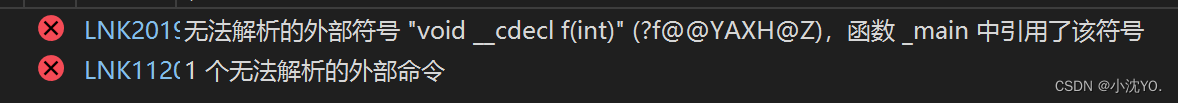``````//Func.h
#define _CRT_SECURE_NO_WARNINGS 1
#include<iostream>

using namespace std;

inline void f(int i);

void fx();

//Func.cpp
#define _CRT_SECURE_NO_WARNINGS 1
#include "Func.h"
void f(int i)
{
cout << "f(int i)" << i << endl;
}
void fx()
{
f(1);//既有声明也有定义这里直接展开不需要地址
}

//Test.cpp
#define _CRT_SECURE_NO_WARNINGS 1
#include<iostream>
#include"Func.h"
using namespace std;
int main()
{
f(1);
fx();
return 0;
}
``````

f()这个函数肯定是在的，不然fx()就不会调到它，但是Test.cpp中的 f() 不可调用Func.cpp中的 f() 可以调用，一般只有声明没有定义调不到，但是在Func.cpp中定义了 f()也调不到，原因就是f()函数定义成了内联，在用的地方就展开了就不需要生成指令建立栈帧把地址放进符号表``````//Func.h
//声明和定义不分离
#define _CRT_SECURE_NO_WARNINGS 1
#include<iostream>

using namespace std;

inline void f(int i);
{
cout << "f(int i)" << i << endl;
}

//Test.cpp
#define _CRT_SECURE_NO_WARNINGS 1
#include<iostream>
#include"Func.h"
using namespace std;
int main()
{
f(1);
return 0;
}
``````

# 🌟二、auto关键字

## 🌏2.1.类型别名思考

1. 类型难于拼写
2. 含义不明确导致容易出错
``````#include<iostream>
#include<vector>
#include<string>
using namespace std;
int TestAuto()
{
return 10;
}
int main()
{
std::vector<std::string>v;
//std::vector<std::string>::iterator it = v.begin();《==》auto it = v.begin();
auto it = v.begin();
cout << typeid(it).name() << endl;
//auto e; 无法通过编译，使用auto定义变量时必须对其进行初始化
return 0;
}
``````

## 🌏2.2.auto简介

C++11中，标准委员会赋予了auto全新的含义即：auto不再是一个存储类型指示符，而是作为一个新的类型指示符来指示编译器，auto声明的变量必须由编译器在编译时期推导而得（根据右边的值自动推导左边的类型）

``````int TestAuto()
{
return 10;
}
int main()
{
int a = 10;
auto b = a;
auto c = 'a';
auto d = TestAuto();
cout << typeid(b).name() << endl;
cout << typeid(c).name() << endl;
cout << typeid(d).name() << endl;
//auto e; 无法通过编译，使用auto定义变量时必须对其进行初始化
return 0;
}
``````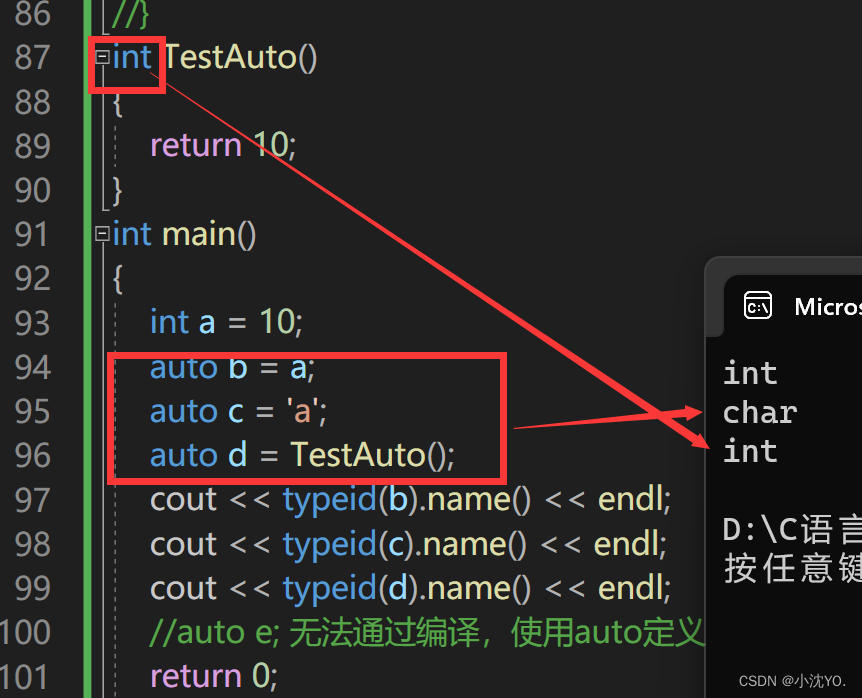## 🌏2.3.auto的使用细节

``````int main()
{
int x = 10;
auto a = &x;
auto* b = &x;
auto& c = x;
cout << typeid(a).name() << endl;
cout << typeid(b).name() << endl;
cout << typeid(c).name() << endl;
return 0;
}
``````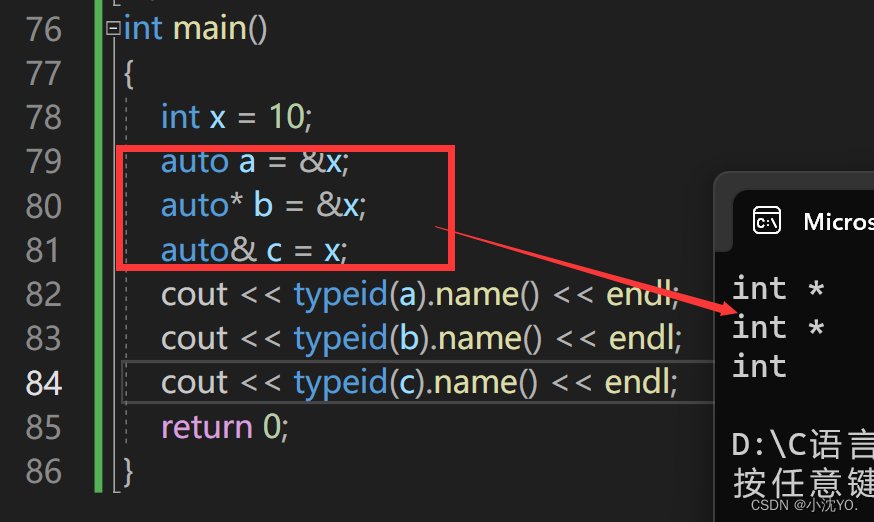``````void TestAuto()
{
auto a = 1, b = 2;
auto c = 3, d = 4.0;  // 该行代码会编译失败，因为c和d的初始化表达式类型不同
}
``````

## 🌏2.4.auto不能推导的场景

1. auto不能作为函数的参数
``````// 此处代码编译失败，auto不能作为形参类型，因为编译器无法对a的实际类型进行推导
void TestAuto(auto a)
{}
``````
1. auto不能直接用来声明数组
``````void TestAuto()
{
int a[] = {1,2,3};
auto b[] = {4，5，6};
}
``````
1. 为了避免与C++98中的auto发生混淆，C++11只保留了auto作为类型指示符的用法
2. auto在实际中最常见的优势用法就是跟以后会讲到的C++11提供的新式for循环，还有lambda表达式等进行配合使用。

## 🌏2.5.小场景补充

TypeId 返回一个变量或数据类型的“类型”。

# 🌟三、基于范围的for循环

## 🌏3.1.范围for的语法

``````#include<iostream>
using namespace std;
void TestFor()
{
int array[] = { 1, 2, 3, 4, 5 };
for (int i = 0; i < sizeof(array) / sizeof(array); ++i)
array[i] *= 2;
for (int* p = array; p < array + sizeof(array) / sizeof(array); ++p)
cout << *p<<" ";
}
int main()
{
TestFor();
return 0;
}
``````

``````#include<iostream>
using namespace std;
void TestFor()
{
int array[] = { 1, 2, 3, 4, 5 };
for (int i = 0; i < sizeof(array) / sizeof(array); ++i)
array[i] *= 2;
for (int* p = array; p < array + sizeof(array) / sizeof(array); ++p)
cout << *p<<" ";
cout << endl;
for (auto& n : array)//至于这里为什么采用引用
//是因为不采用引用只是从array中取数赋值给n，n*=2发生变化对数组没影响所以要引用才能改变数组
{
n *= 2;
}
for (auto m : array)
//当然也不是必须写成auto m,可以int m ,double m,只是auto会根据右边值的类型推导出左边类型
{
cout << m << " ";
}
cout << endl;
}
int main()
{
TestFor();
return 0;
}
``````

## 🌏3.2.范围for的使用条件

begin和end的方法，begin和end就是for循环迭代的范围。

``````void TestFor(int array[])
{
for(auto& e : array)
cout<< e <<endl;
}
``````

# 🌟四、指针空值nullptr

``````void TestPtr()
{
int* p1 = NULL;
int* p2 = 0;
// ……
}
``````

NULL实际是一个宏，在传统的C头文件(stddef.h)中，可以看到如下代码：

``````#ifndef NULL
#ifdef __cplusplus
#define NULL   0
#else
#define NULL   ((void *)0)
#endif
#endif
``````

``````#include<iostream>
using namespace std;
void f(int)
{
cout << "f(int)" << endl;
}
void f(int*)
{
cout << "f(int*)" << endl;
}
int main()
{
f(0);
f(NULL);
f((int*)NULL);
return 0;
}
``````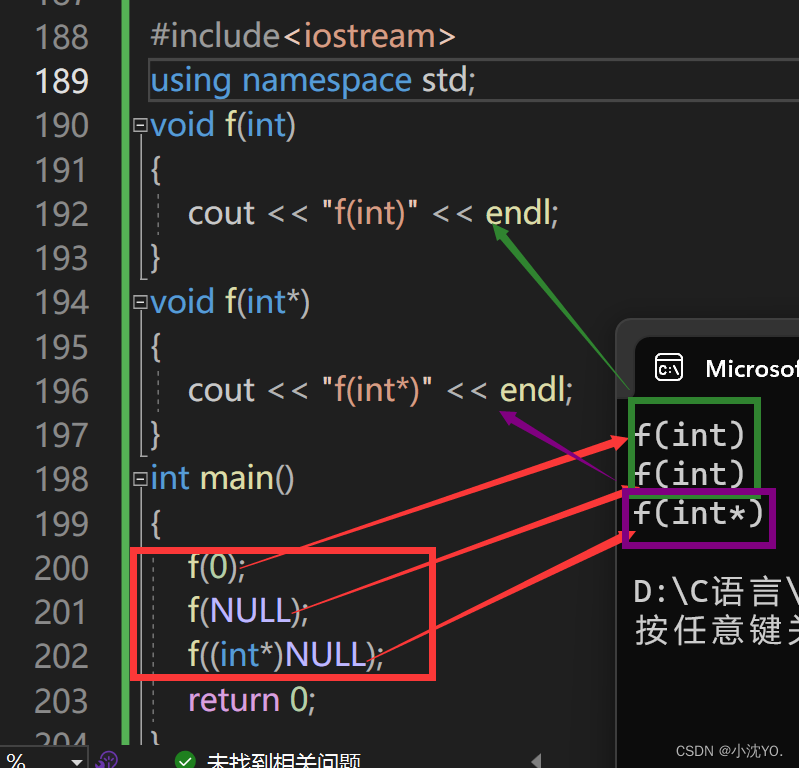• 在使用nullptr表示指针空值时，不需要包含头文件，因为nullptr是C++11作为新关键字引入的。

• 在C++11中，sizeof(nullptr) 与 sizeof((void*)0)所占的字节数相同。

• 为了提高代码的健壮性，在后续表示指针空值时建议最好使用nullptr

### [Linux]进程概念

[Linux]进程概念 文章目录 [Linux]进程概念进程的定义进程和程序的关系Linux下查看进程Linux下通过系统调用获取进程标示符Linux下通过系统调用创建进程-fork函数使用 进程的定义 进程是程序的一个执行实例&#xff0c;是担当分配系统资源&#xff08;CPU时间&#xff0c;内存…

### 3.BGP状态机和路由注入方式

BGP状态机 BGP路由的生成 不同于IGP路由协议，BGP自身并不会发现并计算产生路由，BGP将GP路由表中的路由注入到BGP路由表中，并通过Update报文传递给BGP对等体。 BGP注入路由的方式有两种： Networkimport-route与IGP协议相同，BGP支持根据已有的路由条目进行聚合，生成聚合路由…

### 关于css 的选择器和 css变量

css 选择器 常用的选择器 1. 后代选择器&#xff1a;也就是我们常见的空格选择器&#xff0c;选择的对象为该元素下的所有子元素 。例如&#xff0c;选择所有 元素下的 元素 div p{font-size:14px}2. 子元素选择器 ‘>’ 选择某元素下的直接子元素。例如&#xff0c;选择所…

### stm32之8.中断

&#xff08;Exceptions&#xff09;异常是导致程序流更改的事件&#xff0c;发生这种情况&#xff0c;处理器将挂起当前执行的任务&#xff0c;并执行程序的一部分&#xff0c;称之为异常处理函数。在完成异常处理程序的执行之后&#xff0c;处理器将恢复正常的程序执行&#…

### PyQt open3d 加载 显示点云

PyQt加载 显示点云&#xff0c;已经有三种方式&#xff0c;使用 open3d; 使用 vtk; 使用 pcl; 下面是使用 open3d: import sys import open3d as o3d import numpy as np import pyqtgraph.opengl as gl from PyQt5.QtWidgets import QApplication, QVBoxLayout, QWidget, QFi…